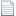# New physics based on new geometryslides.pdf

Deepen our understanding of world is the fundamental goal of High Energy Physicist. Building model, deriving its predictions and searching experimental evidence are major works of High Energy Physicists. As a junior theoretical particle physicist, I build a minimal unified field theory based on square root metric geometry [arXiv:1412.2578 [hep-th]]. This theory is generally covariant, U(4) gauge invariant and unitary. Based on the parallel transport (one fundamental axiom of general relativity), the U(4) Yang-Mills theory in curved space-time and the Einstein-Hilbert action are derived. There are several predictions about new physics as follows:

1.        Higgs mechanism: Higgs mechanism is not the reason to give W and Z mass. In fact, Klein-Gorden equation should not be a fundamental equation because squares of exterior derivatives equals zero (d2=0).

2.        W and Z: W and Z are composite color singlet particles.

3.        Dark matter: The U(4) Yang-Mills theory has the U(1) conserved current and I call it Fiona (dark photon). Fiona almost not interacts with electro-magnetism, weak and strong but strongly interacts with gravity.

4.        Spacetime: The spacetime is described by square root Riemannian geometry, not Lorentzian manifold.

5.        Neutrino: Right handed neutrinos should exist.

http://blog.sciencenet.cn/blog-319569-1164084.html

## 全部精选博文导读

GMT+8, 2019-8-25 06:38

Powered by ScienceNet.cn

Copyright © 2007- 中国科学报社